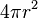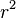Kids Encyclopedia Facts

The steradian (symbol: sr) or square radian is an SI unit. It is like a radian, but for smooth 3D objects, for example, a sphere. A steradian is about one twelfth the surface area of a sphere, regardless of how big the sphere is.

• The surface area of a sphere is$4\pi r^2$
• The surface area of a steradian is just$r^2$In Spanish: Estereorradián para niños